## Thursday, October 13, 2016

### Math Family Facts – Multiply and Divide by 11

These slides feature multiplying and dividing by 11 by the use of math family facts, demonstrating the commutative property for multiplication. This is part of a series in which numbers one through twelve are multiplied and divided by one through twelve. Each number is multiplied by zero but not divided by zero.

These serve as a good review of information in which readers can look at patterns. The numbers are highlighted in different colors to make it easier to see how they are applied in the math equations.

Directions
1. Click on the first slide to enlarge.
2. Click on the slide or thumbnail to advance to the next slide.

Find more than 300 free resources at my Student Survive 2 Thrive blog. Thanks for visiting, and feel free to post comments in the comment section below the last slide.Math Family Facts - Multiply and Divide by 11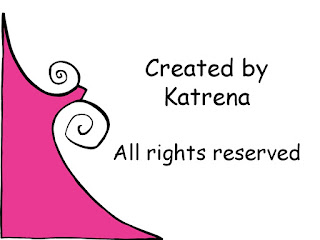Free Math Resource by Katrena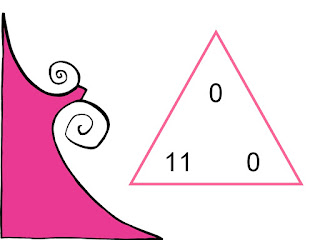Math Family Facts for 11 and 0 and 011 X 0 = 1, 0 X 11 = 0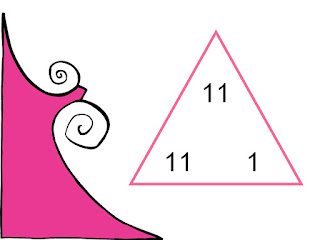Math Family Fact for 11 and 1 and 11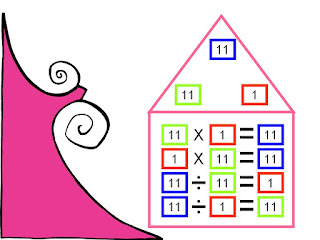11 X 1 = 11, 1 X 11 = 11, 11 Divided by 11 = 1, 11 Divided by 1 = 11Math Family Fact for 11 and 2 and 22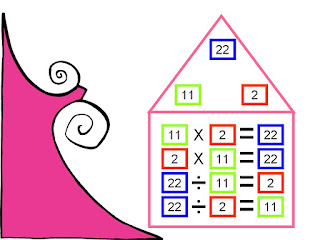11 X 2 = 22, 2 X 11 = 22, 22 Divided by 11 = 2, 22 Divided by 2 = 11Math Family Fact for 11 and 3 and 3311 X 3 = 33, 3 X 11 = 33, 33 Divided by 11 = 3, 33 Divided by 3 = 11Math Family Facts for 11 and 4 and 4411 X 4 = 44, 4 X 11 = 44, 44 Divided by 11 = 4, 44 Divided by 4 = 11Math Family Facts for 11 and 5 and 5511 X 5 = 55, 5 X 11 = 55, 55 Divided by 11 = 5, 55 Divided by 5 = 11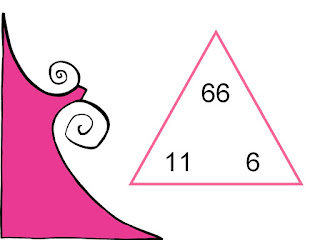Math Family Facts for 11 and 6 and 6611 X 6 = 66, 6 X 11 = 66, 66 Divided by 11 = 6, 66 Divided by 6 = 11Math Family Facts for 11 and 7 and 7711 X 7 = 77, 7 X 11 = 77, 77 Divided by 11 = 7, 77 Divided by 7 = 11Math Family Facts for 11 and 8 and 88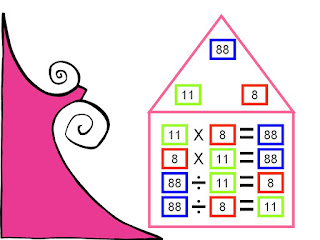11 X 8 = 88, 8 X 11 = 88, 88 Divided by 11 = 8, 88 Divided by 8 = 11Math Family Facts for 11 and 9 and 99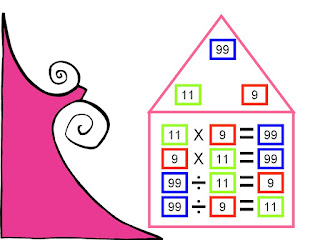11 X 9 = 99, 9 X 11 = 99, 99 Divided by 11 = 9, 99 Divided by 9 = 11Math Family Facts for 11 and 10 and 11011 X 10 = 110, 10 X 11 = 110, 110 Divided by 11 = 10, 110 Divided by 10 = 11Math Family Facts for 11 and 11 and 12111 X 11 = 121, 121 Divided by 11 = 11Math Family Facts for 11 and 12 and 132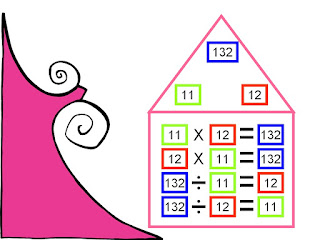11 X 12 = 132, 12 X 11 = 132, 132 Divided by 11 = 12, 132 Divided by 12 = 11Free Math Resource at Student Survive 2 Thrive